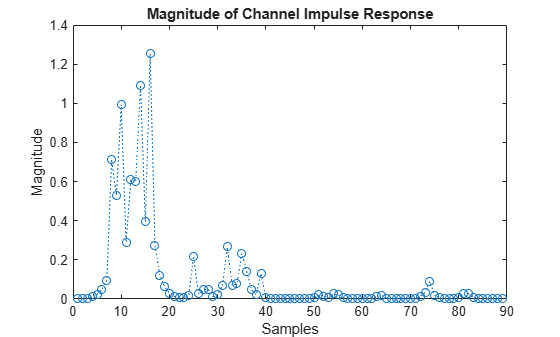# getPathFilters

Get path filter impulse response for 3-D MIMO fading channel

## Syntax

``pathFilters = getPathFilters(lte3d)``

## Description

example

````pathFilters = getPathFilters(lte3d)` returns path filter impulse responses for the 3-D multi-input/multi-output (MIMO) fading channel object specified by `lte3d`. You can use this information, along with the `pathGains` output argument returned by the channel object, to reconstruct a perfect channel estimate.```

## Examples

collapse all

Reconstruct the channel impulse response and perform timing offset estimation using path filters of an `lte3DChannel` System object.

Configure a channel for delay profile CDL-B from TR 38.901 Section 7.7.1, with 1000 ns delay spread, 2 transmit antennas, and 1 receive antenna.

```lte3d = lte3DChannel.makeCDL('CDL-B',1000e-9); lte3d.Seed = 11; lte3d.TransmitAntennaArray.Size = [1 1 2]; lte3d.ReceiveAntennaArray.Size = [1 1 1];```

Create an LTE waveform for reference measurement channel (RMC) R.10 (10MHz, QPSK, R=1/3, 2 CRS ports).

```rmc = lteRMCDL('R.10'); rmc.TotSubframes = 1; data = [1; 0; 0; 1]; [txWaveform,~,txInfo] = lteRMCDLTool(rmc,data); lte3d.SampleRate = txInfo.SamplingRate;```

Pass the waveform through the channel.

`[rxWaveform,pathGains] = lte3d(txWaveform);`

Perform timing offset estimation using cell-specific reference signals.

```corrcfg.CellRS = 'On'; offset = lteDLFrameOffset(rmc,rxWaveform,corrcfg)```
```offset = 15 ```

Obtain the path filters used in channel filtering.

`pathFilters = getPathFilters(lte3d);`

Reconstruct the channel impulse response using the path filters and path gains. Take the average of path gains across all time samples (first dimension). Construct the impulse response, h, for each transmit and receive antenna. Sum the responses for each transmit antenna.

```[~,Np,P,R] = size(pathGains); Nh = size(pathFilters,1); h = zeros([Nh P R]); pathGains = permute(mean(pathGains,1),[2 3 4 1]); for np = 1:Np h = h + pathFilters(:,np) .* pathGains(np,:,:); end h = permute(sum(h,2),[1 3 2]); mag = abs(h);```

Plot the magnitude of the channel impulse response.

```plot(mag,'o:') title('Magnitude of Channel Impulse Response') xlabel('Samples') ylabel('Magnitude')```Estimate the timing offset by finding the peak of the impulse response magnitude.

`offset_ref = find(mag==max(mag)) - 1`
```offset_ref = 15 ```

## Input Arguments

collapse all

MIMO fading channel, specified as an `lte3DChannel` System object. This object implements the TR 36.873 link-level MIMO fading channel.

## Output Arguments

collapse all

Path filter impulse response, returned as an Nh-by-Np real matrix, where:

• Nh is the number of impulse response samples.

• Np is the number of paths.

Each column of the matrix contains the filter impulse response for each path of the delay profile.

Data Types: `double`

## Version History

Introduced in R2018a